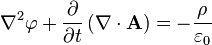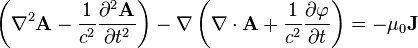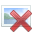# Maxwell equations in terms of potentials

• olgerm

#### olgerm

Gold Member
These are Maxwell´s equations in potential formulation:2φ = DIV(grad(φ)) . Am I right?
2A = ROT(ROT(A))=ROT(B)=grad(DIV(A))-Laplace(A) . Am I right?
In coulomb gauge in every point and at any time DIV(A)=[PLAIN]https://upload.wikimedia.org/math/4/4/1/44131cc26bd9db464d0edb7459ccca84.png. [Broken] Am I right?
Where could I find Maxwell´s equations in terms of potentials without vector operator?

How must ROT (same as curl) be generalized to make the equations describe EM-field in D-dimensional space equally with these equation
##\begin{cases}
& \sum_{i=1}^D(\frac{\partial E_i}{\partial x_i})=\rho \frac{1}{{\epsilon_0}} \\
& \frac{\partial E_a}{\partial t}=\sum_{i=1}^D(\frac{\partial B_{[i;a]}}{\partial x_i})-J_a \\
& \frac{\partial B_{[a;b]}}{\partial t}=\frac{\partial E_b}{\partial x_a}-\frac{\partial E_a}{\partial x_b}\\
& \frac{\partial B_{[a;b]}}{\partial x_c}+\frac{\partial B_{[b;c]}}{\partial x_a}+\frac{\partial B_{[c;a]}}{\partial x_b}=0
\end{cases}##
,which are in terms of E and B?

φ is electripotentialfield.
E is electricvectorfield.
A is magneticpotentialvectorfield.
B is magneticvectorfield.
ρ is electriccharge density.

Last edited by a moderator:

∇2φ = DIV(grad(φ)) . Am I right?
Yes
∇2A = ROT(ROT(A))=ROT(B)=grad(DIV(A))-Laplace(A) . Am I right?
No. Look here: https://en.wikipedia.org/wiki/Vector_Laplacian
In coulomb gauge in every point and at any time DIV(A)=. Am I right?
Yes
Where could I find Maxwell´s equations in terms of potentials without vector operator?
You can do this yourself. Just take what you have above and replace with partial derivatives. Also, this might be useful
https://en.wikipedia.org/wiki/Covariant_formulation_of_classical_electromagnetism

Your last question is a little weird. Are interested in a 2D subset (x,y) of 3D space (x,y,z) where z is a symmetry?

•olgerm
It seems to confirm, that ROT(ROT(A))=grad(DIV(A))-Laplace(A) .
also this http://m.wolframalpha.com/input/?i=curl+(curl+A)&x=0&y=0 seems to confirm, that ROT(ROT(A))=grad(DIV(A))-Laplace(A) . Or does ∇2 A in the second equation note Laplace(A) not ROT(ROT(A))?

Your last question is a little weird. Are interested in a 2D subset (x,y) of 3D space (x,y,z) where z is a symmetry?
I am interested about theoretical physical system with D-dimensional space. Understanding physical meaning of such system is not necessary to answer the question.

##\nabla^2 A## means the same thing as Laplace(A).

How many dimensions do you want to work in?

•olgerm
How many dimensions do you want to work in?
D dimensions. Generalization must include variable D.

Now as I understand there are different Laplacians for vectorfield and scalarfield it makes more sense.

So are these equations in lorenz gauge
##\begin{cases}
\frac{\partial^2 A_{D_1}}{\partial t^2}=J_{D_1}\cdot k_E \cdot \pi \cdot c^2 \cdot 4+\sum_{i=1}^D(\frac{\partial^2 A}{\partial x_i \cdot \partial x_{D_1}}) \cdot c^2-ROT(ROT(A)) \cdot c^2\\
\frac{\partial^2 \phi}{\partial t^2}=\rho \cdot k_E \cdot \pi \cdot 4+\sum_{i=1}^D(\frac{\partial^2 \phi}{\partial x_i^2})\\
\frac{\partial \phi}{\partial t}=\sum_{i=1}^D(\frac{\partial A_i}{\partial x_i}) \cdot c^2\\
F_E=q\cdot(\sum_{i=1}^D(v_i \cdot \frac{\partial A_i}{\partial x_{D_1}})-\frac{\partial A_{D_1}}{\partial t}-\frac{\partial \phi}{\partial x_{D_1}})
\end{cases}##
?

But what must ROT(ROT(A)) equal to in D-dimensional space so that the Maxwell equations were equal with these
##\begin{cases}
& \sum_{i=1}^D(\frac{\partial E_i}{\partial x_i})=\rho \frac{1}{{\epsilon_0}} \\
& \frac{\partial E_a}{\partial t}=\sum_{i=1}^D(\frac{\partial B_{[i;a]}}{\partial x_i})-J_a \\
& \frac{\partial B_{[a;b]}}{\partial t}=\frac{\partial E_b}{\partial x_a}-\frac{\partial E_a}{\partial x_b}\\
& \frac{\partial B_{[a;b]}}{\partial x_c}+\frac{\partial B_{[b;c]}}{\partial x_a}+\frac{\partial B_{[c;a]}}{\partial x_b}=0
\end{cases}##
equation?
I also want the equation system to be formed as short and easy as possible. What gauge you recommend me to choose? Any ideas how to simplify the equations?

Last edited:
I have hypothesis, that Laplace(A) must be generalized to be equal to ## 2\cdot\frac{\partial^2 A_{D_1}}{\partial x_{D_1}^2}-\sum_{i=1}^D(\frac{\partial^2 A_{D_1}}{\partial x_i^2})## .
Can anybody check if it is correct?

You know what the electromagnetic tensor is? You should use a tensor formulation if you want to generalize to different dimensions. I asked what dimension you wanted to work in because it affects the answer. Cross product only really works in 3 or 7 dimensions. You might want to use the Levi-Civita symbol.

You know what the electromagnetic tensor is? You should use a tensor formulation if you want to generalize to different dimensions.
I got the equations in E and B formulation from Maxwell equations in electromagnetic tensor formulation.

The equations should not contain vector products on operators, but partial derivatives and sums. D must be a variabe, that can be equal to any natural number.

Last edited:
I have got my answer. Correct equation system is:
##\begin{cases}
\frac{\partial^2 A_{D_1}}{\partial t^2}=J_{D_1}\cdot k_E \cdot \pi \cdot 4+\sum_{i=1}^D(\frac{\partial^2 A_{D_1}}{\partial x_i^2}) \cdot c^2\\
\frac{\partial^2 \phi}{\partial t^2}=\rho \cdot k_E \cdot c^2 \cdot \pi \cdot 4+\sum_{i=1}^D(\frac{\partial^2 \phi}{\partial x_i^2})\\
\frac{\partial \phi}{\partial t}=\sum_{i=1}^D(\frac{\partial A_i}{\partial x_i}) \cdot c^2\\
F_E=q\cdot(\sum_{i=1}^D(v_i \cdot \frac{\partial A_i}{\partial x_{D_1}})-\frac{\partial A_{D_1}}{\partial t}-\frac{\partial \phi}{\partial x_{D_1}})
\end{cases}##